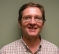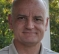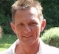## Problem with natural logarithmic function in fixed point notationStarted by 3 years ago17 replieslatest reply 3 years ago373 views

I am facing a problem with natural logarithmic function in fixed-point notation.

Let's say

x = 0.54, then ln(x) = -0.616186, a negative value => (1)

Then in fixed-point notation, the value of x is calculated by (assuming x is Q8.24 format)

temp_var = 0.54 * 16777216 = 9059696 (fixed point value in Q8.24 format)

So,

x = ln(temp_var) = 16.01 (since 2^24 = 16777216)

And the float (true / unscaled form) value corrosponds to

16.01 / 16777216 = 9.54e-7, a positive value => (2)

As we can see, both (1) and (2) are different in both magnitude and sign.

So, I just want to know how to solve these kinds of problems at a fixed point?

[ - ]as mentioned by @bholzmayer you may need to do sub instead of div.

the sample calculation may help:

x =  0.54000.

log(x) = -0.61619                      ----(1)

xx  = x*2^24  =  9059696.64000

log(xx) = 16.019

log(x) = log(xx)-log(2^24)= -0.61619   ----(2)

hope it helps.

-chalil

[ - ]Hi chalil,

I am not facing any problems in computing log(x) or x. The problem is that the float (true) value of x is different from the value of x, computed after doing fixed point math.

I was told that i need to add some constant negative offset to the output obtained in fixed point math. It seems that i need to add that constant value before doing division operation, in order to solve the problem i am facing. However, now, i am not able to understand how to calculate the constant negative offset.

[ - ]looks like you are talking about something very specific. I'm attaching one sample code which computes fixed point log(x). it uses simple curve fit approach and the range of x you can give here is between 0.1 and 1.0.

you can build the code with GCC or any c compiler. when you run it gives you the fixed point output (scaled back to float range), the float point computed with the same polinomial fit and the floating point log(x) out. for x = 0.54

Value based on log_fix = -0.618589

Value based on log_flt = -0.618589

Value based on log_ref = -0.616186

you can see one and two are very close and even the same for the displayed precision.

the difference between 2 with 3 can be improved by better curve fit or any other approach.

log_fit.c

[ - ]I recently coded up an RSSI dB function for a receiver implemented in an FPGA.  Not quite what you are doing here but the concept is the same.  I am finding 20*log10 of a 19-bit fixed point number.

1st I find the number of leading zeros.  This would normally be a log2 but of course I am scaling it all to dB so it is just a lookup value.  Also I left shift the remaining data to the non-zero part.

2nd I use a small lookup table of the next few bits to find the rest of the dB value to add in.

The method could scale to find the log on any base.

For an AGC loop I have done a log2 approximation by taking the leading zeros as the whole part and the bits after the 1 as the fractional part.  It worked very well and is simple to implement in logic.

FWIW

[ - ]Hi napierm,

I went through almost the exact same idea several years ago for  measuring signal power in an audio application.

The only thing I would mention that relates to what you said, is that 20*log10(x) = 6.02*log2(x), and similarly, 10*log10(x) = 3.01*log2(x).

In each case, the 6.02 or 3.01 can be approximated reasonably well with a simple shift and add pair, with an error of 0.3%, which is probably less than the error you left based on the size of your lookup table.

[ - ]Yes that would work fine.  And honestly 0.3% error is never going to be seen in a noise measurement.

[ - ]Post deleted by author

[ - ]If you are really stuck, and have only paper and pencil in hand...and some free time: A Recipe for a Common Logarithm Table

[ - ]This may help

y1 = log(0.54)

e^log(y1)

y2 = log(0.54*2^24)

e^y2/2^24

[ - ]Hi, i didn't understand your logic. Can you explain in detail?

[ - ]If you started with log(0.54) you get some result.

if you scale 0.54 by 2^24 you get a large number and a new log.

Thus you need to scale down that result...so what is the problem that you see?

[ - ]if:  y=x*(2^24)

then: ln(y)=ln(x)+ln(2^24)

results in your case, where x=0.54 to

y=ln(x)+ln(2^24)=-0.616.. + 16.63..=16.01

[ - ]Hi, i have mentioned the similar calculation in the query itself. And moreover, calculating the value of x in fixed point is not my query.

[ - ]If values are multiplied (divided), you have to add (subtract) their logarithms to get the same result.

This is applying too, if you use fixed point scaling since it's multiplying (dividing) values with the scale.

you get ln(temp_var) = ln(x) + ln(16777216)

I cannot see this in your query...

[ - ]Hi,

I think, we can do the calculations in both the ways.

1st Method: ln(0.54 * 16777216) = ln(9059696) = 16.01

2nd Method: ln(0.54 * 16777216) = ln(0.54) + ln(16777216)

= -0.616186 + 16.635532 = 16.01

Both methods are giving same results. So, i opted for 1st method (direct method). Please correct me if i am wrong.

[ - ]x = 0.54   and ln(x) = -0.616186         ==> 1

x = (0.54 * 2^24) / (2^24)

Taking natural log on both sides,

ln(x) = ln(0.54 * 2^24) - ln(2^24)

= 16.019346194 - 16.6355323334

= -0.616186                        ==> 2

As @bholzmayer replied, you are dividing ln(2^24) instead subtracting.

[ - ]It may help you conceptually to view the same situation in common logarithms and scientific notation.

For instance:

log(2) ~=~ 0.301

log(200) ~=~ 2.301

log(0.02) ~=~ -1.699

Now, in Scientific notation

log(2x10^0) ~=~    0.301

log(2x10^2) ~=~    0.301 + 2

log(2x10^{-2}) ~=~ 0.301 - 2

Indeed, when you are working these kinds of problems by hand (pre calculator/computer days) you carried and worked with the components separated as that made the math easier over all.  Yep, I'm old enough.  Log tables only go from 1.0 to 10.0 which is decimal done in fixed point.

The words are "mantissa" and "exponent".

log(A*10^B) = log(A) + log(10^B) = log(A) + B*log(10) = log(A) + B

B represents decimal shifting.

The same principles apply in base 2 like implemented in IEEE floating point values.

There is no numbering system corresponding to natural logs so it begs the question, why are you mixing binary fixed point with natural logs and not using base 2 logs instead?